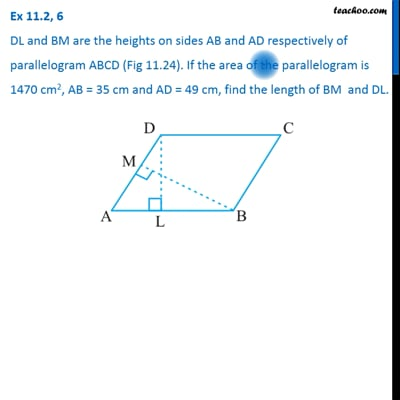Area of parallelogram

Chapter 9 Class 7 Perimeter and Area
Concept wiseThis video is only available for Teachoo black users

Get live Maths 1-on-1 Classs - Class 6 to 12

### Transcript

Ex 9.1, 6 DL and BM are the heights on sides AB and AD respectively of parallelogram ABCD (Fig 11.24). If the area of the parallelogram is 1470 cm2, AB = 35 cm and AD = 49 cm, find the length of BM and DL. Here, We can find area of parallelogram using: AB as base & DL as height Or AD as base & BM as height Given, Area = 1470 cm2 Base = AB = 35 cm Height = DL = ? Area of parallelogram = Base × Height 1470 = AB × DL 1470 = 35 × DL 1470/35 = DL 210/5 = DL 42 = DL DL = 42 cm 1470/35 = DL 210/5 = DL 42 = DL DL = 42 cm Given, Area = 1470 cm2 Base = AB = 35 cm Height = DL = ? Area of parallelogram = Base × Height 1470 = AB × DL 1470 = 35 × DL Using AD & BM Given, Area = 1470 cm2 Base = AD = 35 cm Height = BM = ? Area of parallelogram = Base × Height 1470 = AD × BM 1470 = 49 × BM 1470/35 = DL 210/5 = DL 42 = DL DL = 42 cm 1470/49 = BM 210/7 = BM 30 = BM BM = 30 cm ∴ Length of DL is 42 cm and Length of BM is 30 cm